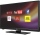# Fraction calculator

The calculator performs basic and advanced operations with fractions, expressions with fractions combined with integers, decimals, and mixed numbers. It also shows detailed step-by-step information about the fraction calculation procedure. Solve problems with two, three, or more fractions and numbers in one expression.

## Result:

### 35/6 + 21/4 = 73/12 = 6 1/12 ≅ 6.0833333

Spelled result in words is seventy-three twelfths (or six and one twelfth).

### How do you solve fractions step by step?

1. Conversion a mixed number 3 5/6 to a improper fraction: 3 5/6 = 3 5/6 = 3 · 6 + 5/6 = 18 + 5/6 = 23/6

To find new numerator:
a) Multiply the whole number 3 by the denominator 6. Whole number 3 equally 3 * 6/6 = 18/6
b) Add the answer from previous step 18 to the numerator 5. New numerator is 18 + 5 = 23
c) Write a previous answer (new numerator 23) over the denominator 6.

Three and five sixths is twenty-three sixths
2. Conversion a mixed number 2 1/4 to a improper fraction: 2 1/4 = 2 1/4 = 2 · 4 + 1/4 = 8 + 1/4 = 9/4

To find new numerator:
a) Multiply the whole number 2 by the denominator 4. Whole number 2 equally 2 * 4/4 = 8/4
b) Add the answer from previous step 8 to the numerator 1. New numerator is 8 + 1 = 9
c) Write a previous answer (new numerator 9) over the denominator 4.

Two and one quarter is nine quarters
3. Add: 23/6 + 9/4 = 23 · 2/6 · 2 + 9 · 3/4 · 3 = 46/12 + 27/12 = 46 + 27/12 = 73/12
For adding, subtracting, and comparing fractions, it is suitable to adjust both fractions to a common (equal, identical) denominator. The common denominator you can calculate as the least common multiple of both denominators - LCM(6, 4) = 12. In practice, it is enough to find the common denominator (not necessarily the lowest) by multiplying the denominators: 6 × 4 = 24. In the next intermediate step, the fraction result cannot be further simplified by canceling.
In words - twenty-three sixths plus nine quarters = seventy-three twelfths.

#### Rules for expressions with fractions:

Fractions - use the slash “/” between the numerator and denominator, i.e., for five-hundredths, enter 5/100. If you are using mixed numbers, be sure to leave a single space between the whole and fraction part.
The slash separates the numerator (number above a fraction line) and denominator (number below).

Mixed numerals (mixed fractions or mixed numbers) write as non-zero integer separated by one space and fraction i.e., 1 2/3 (having the same sign). An example of a negative mixed fraction: -5 1/2.
Because slash is both signs for fraction line and division, we recommended use colon (:) as the operator of division fractions i.e., 1/2 : 3.

Decimals (decimal numbers) enter with a decimal point . and they are automatically converted to fractions - i.e. 1.45.

The colon : and slash / is the symbol of division. Can be used to divide mixed numbers 1 2/3 : 4 3/8 or can be used for write complex fractions i.e. 1/2 : 1/3.
An asterisk * or × is the symbol for multiplication.
Plus + is addition, minus sign - is subtraction and ()[] is mathematical parentheses.
The exponentiation/power symbol is ^ - for example: (7/8-4/5)^2 = (7/8-4/5)2

#### Examples:

subtracting fractions: 2/3 - 1/2
multiplying fractions: 7/8 * 3/9
dividing Fractions: 1/2 : 3/4
exponentiation of fraction: 3/5^3
fractional exponents: 16 ^ 1/2
adding fractions and mixed numbers: 8/5 + 6 2/7
dividing integer and fraction: 5 ÷ 1/2
complex fractions: 5/8 : 2 2/3
decimal to fraction: 0.625
Fraction to Decimal: 1/4
Fraction to Percent: 1/8 %
comparing fractions: 1/4 2/3
multiplying a fraction by a whole number: 6 * 3/4
square root of a fraction: sqrt(1/16)
reducing or simplifying the fraction (simplification) - dividing the numerator and denominator of a fraction by the same non-zero number - equivalent fraction: 4/22
expression with brackets: 1/3 * (1/2 - 3 3/8)
compound fraction: 3/4 of 5/7
fractions multiple: 2/3 of 3/5
divide to find the quotient: 3/5 ÷ 2/3

The calculator follows well-known rules for order of operations. The most common mnemonics for remembering this order of operations are:
PEMDAS - Parentheses, Exponents, Multiplication, Division, Addition, Subtraction.
BEDMAS - Brackets, Exponents, Division, Multiplication, Addition, Subtraction
BODMAS - Brackets, Of or Order, Division, Multiplication, Addition, Subtraction.
GEMDAS - Grouping Symbols - brackets (){}, Exponents, Multiplication, Division, Addition, Subtraction.
Be careful, always do multiplication and division before addition and subtraction. Some operators (+ and -) and (* and /) has the same priority and then must evaluate from left to right.

## Fractions in word problems:3 3/4 + 2 3/5 + 5 1/2 Show your solution.Why does 1 3/4 + 2 9/10 equal 4.65? How do you solve this?2 and 1/8th plus 1 and 1/3rd =Add two mixed fractions: 2 4/6 + 1 3/6Add this two mixed numbers: 1 5/6 + 2 2/11=What is 4 1/2+2/7-213/14?To three-eighths of one third, we add five quarters of one half and multiply the sum by four. How much will we get?Ann ate a third of a pizza and then another quater. Total part of pizza eaten by Ann and how much pizza is left?7 is added to the sum of 4/5 and 6/7How many hours the Andersons watched TV in all Wednesday 3/1 hr Thursdays 2/3 hr Friday 4/5 hr Saturday 3/4 hrWhat is the sum of 2/3+3/5?Find a fraction equivalent to the recurring decimal? 0.435643564356Evaluate expression using BODMAS rule: 1 1/4+1 1/5÷3/5-5/8